Crypto News

# Kraken Daily Market Report for March 30 2021

## Overview

• Total spot trading volume at $1.19 billion, below the 30-day average of$1.39 billion.
• Total futures notional at $456.3 million. • The top five traded coins were, respectively, Bitcoin, Tether, Ethereum, Cardano, and USD Coin. • Strong returns from Aragaon (+26%) and Filecoin (+18%). March 30, 2021$1.19B traded across all markets today
Crypto, EUR, USD, JPY, CAD, GBP, CHF, AUD
XBT
$58721. ↑1.9%$517.6M
USDT
$1.0003 ↑0.05%$216.3M
ETH
$1841.6 ↑1.1%$164.0M
ADA
$1.2118 ↑0.8%$40.8M
USDC
$0.9999 ↑0.0%$30.9M
DOT
$33.972 ↓1.2%$29.6M
XRP
$0.5626 ↓0.6%$23.9M
STORJ
$2.9653 ↑7.6%$19.4M
FIL
$149.42 ↑18%$18.5M
LTC
$195.77 ↑0.8%$17.5M
KSM
$540.73 ↓1.2%$14.1M
LINK
$27.826 ↓1.0%$10.6M
XTZ
$4.5603 ↓1.1%$8.52M
ALGO
$1.3239 ↓5.9%$7.98M
MANA
$1.0881 ↓0.5%$7.65M
UNI
$28.553 ↓1.7%$7.21M
FLOW
$32.946 ↑0.7%$7.09M
ANT
$11.738 ↑26%$7.08M
BCH
$525.02 ↑1.2%$6.69M
SC
$0.0253 ↑1.1%$6.41M
MLN
$101.06 ↓7.7%$5.64M
XLM
$0.4008 ↓0.9%$5.59M
XMR
$244.13 ↑4.3%$5.27M
XDG
$0.0540 ↓0.02%$5.18M
GRT
$1.7633 ↓0.7%$4.09M
AAVE
$377.10 ↑4.3%$4.06M
ATOM
$19.971 ↓1.6%$4.03M
ICX
$2.6783 ↑4.4%$4.02M
LSK
$5.9249 ↑8.6%$4.0M
CRV
$3.2890 ↓6.6%$3.28M
TRX
$0.0660 ↑1.9%$3.19M
OMG
$6.6240 ↓2.5%$3.13M
REP
$40.694 ↑6.4%$3.01M
ZEC
$154.75 ↑3.6%$2.85M
REPV2
$41.538 ↑6.4%$2.53M
OXT
$0.7229 ↑0.9%$2.36M
BAT
$1.1723 ↑2.9%$2.32M
DASH
$216.20 ↑0.5%$2.24M
EOS
$4.3377 ↑1.3%$2.21M
OCEAN
$1.5092 ↓2.3%$2.06M
KAVA
$6.5131 ↑9.7%$2.02M
QTUM
$8.7716 ↑0.01%$2.02M
DAI
$1.0008 ↑0.01%$2.0M
WAVES
$12.314 ↓1.1%$1.68M
COMP
$402.49 ↓2.1%$1.52M
KEEP
$0.7292 ↑2.3%$1.21M
SNX
$17.556 ↓1.4%$1.15M
YFI
$35516. ↑0.2%$1.12M
NANO
$4.8519 ↑1.1%$1.04M
PAXG
$1697.4 ↓1.6%$1.02M
KNC
$2.7983 ↑1.3%$825K
ETC
$13.007 ↑2.9%$627K
EWT
$16.326 ↓1.8%$562K
BAL
$56.864 ↑2.4%$540K
GNO
$151.38 ↑1.8%$229K
TBTC
$59545. ↑0.9%$52.2K

#####################. Trading Volume by Asset. ##########################################

## Trading Volume by Asset

The figures below break down the trading volume of the largest, mid-size, and smallest assets. Cryptos are in purple, fiats are in blue. For each asset, the chart contains the daily trading volume in USD, and the percentage of the total trading volume. The percentages for fiats and cryptos are treated separately, so that they both add up to 100%.

Figure 1: Largest trading assets: trading volume (measured in USD) and its percentage of the total trading volume (March 30 2021)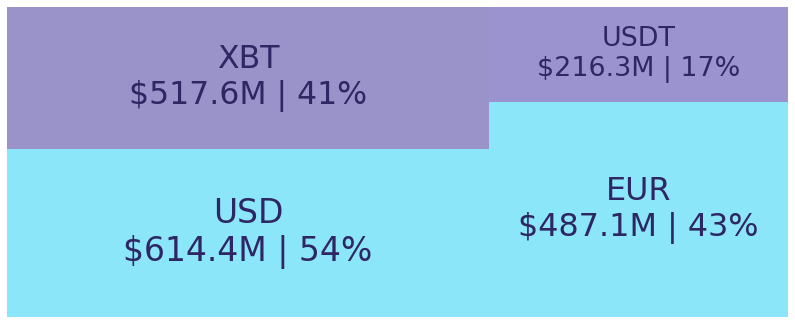Figure 2: Mid-size trading assets: (measured in USD) (March 30 2021)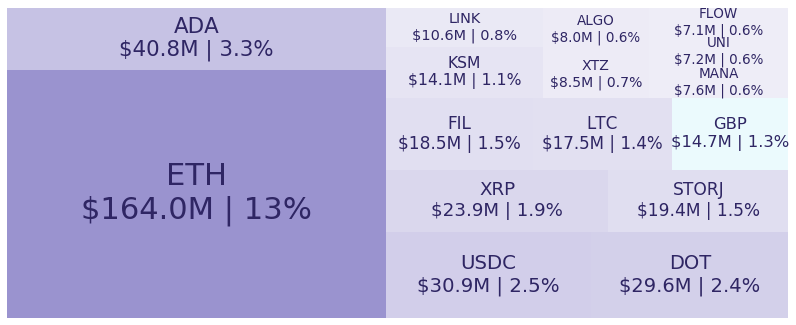Figure 3: Smallest trading assets: (measured in USD) (March 30 2021)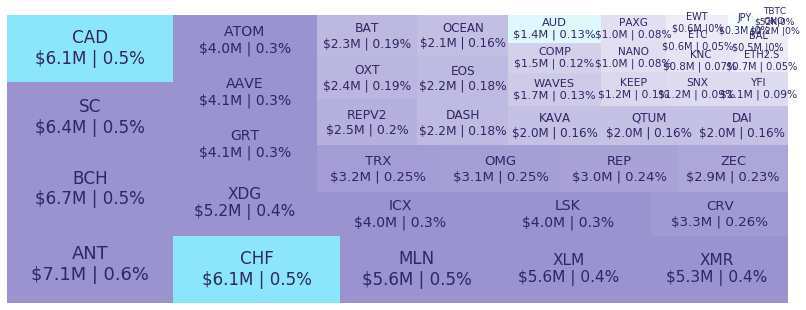#####################. Spread %. ##########################################

## Spread %

Spread percentage is the width of the bid/ask spread divided by the bid/ask midpoint. The values are generated by taking the median spread percentage over each minute, then the average of the medians over the day.

Figure 4: Average spread % by pair (March 30 2021)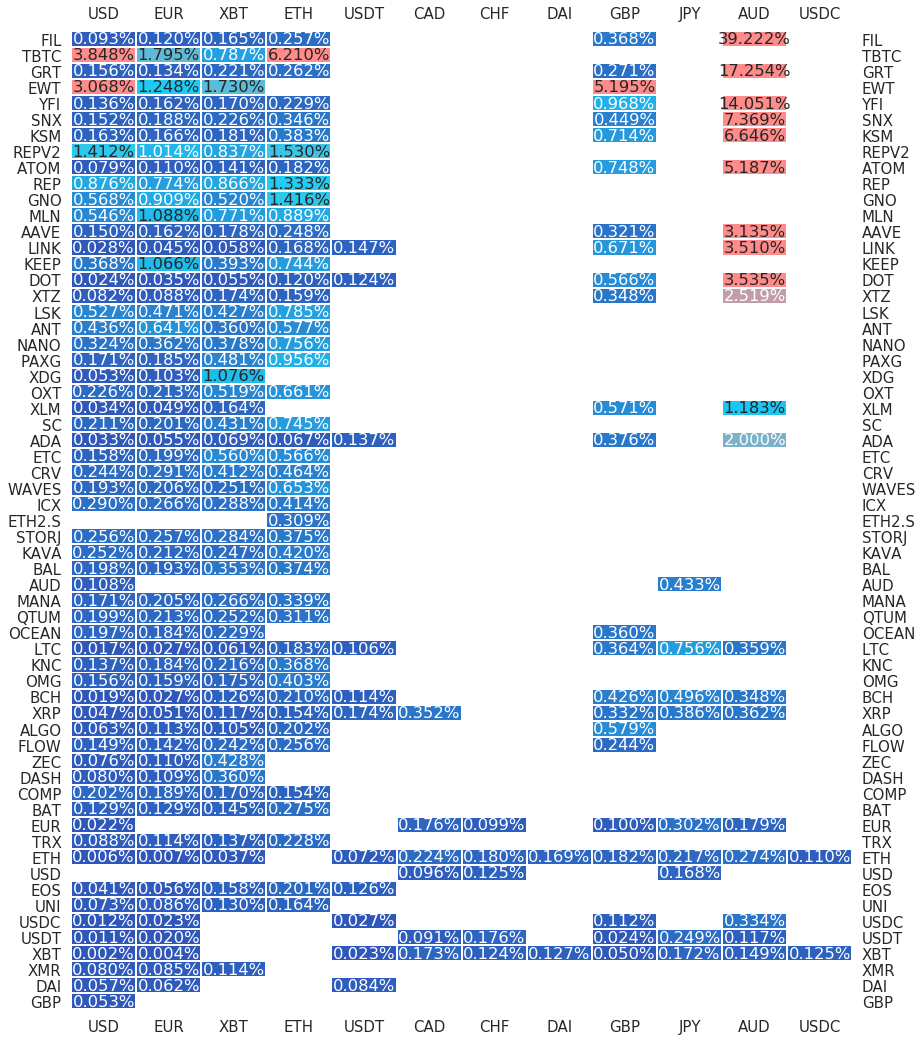.

#########. Returns and Volume ############################################

## Returns and Volume

Figure 5: Returns of the four highest volume pairs (March 30 2021)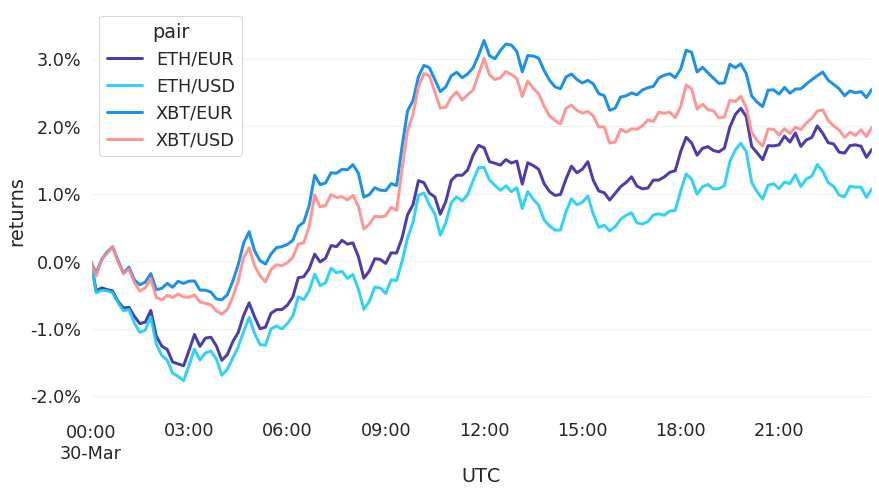Figure 6: Volume of the major currencies and an average line that fits the data to a sinusoidal curve to show the daily volume highs and lows (March 30 2021)